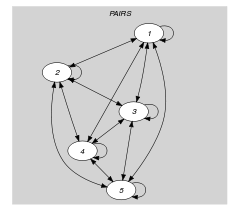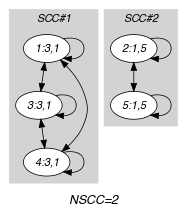## 5.284. npair

Origin

Derived from $\mathrm{𝚗𝚟𝚊𝚕𝚞𝚎}$.

Constraint

$\mathrm{𝚗𝚙𝚊𝚒𝚛}\left(\mathrm{𝙽𝙿𝙰𝙸𝚁𝚂},\mathrm{𝙿𝙰𝙸𝚁𝚂}\right)$

Arguments
 $\mathrm{𝙽𝙿𝙰𝙸𝚁𝚂}$ $\mathrm{𝚍𝚟𝚊𝚛}$ $\mathrm{𝙿𝙰𝙸𝚁𝚂}$ $\mathrm{𝚌𝚘𝚕𝚕𝚎𝚌𝚝𝚒𝚘𝚗}\left(𝚡-\mathrm{𝚍𝚟𝚊𝚛},𝚢-\mathrm{𝚍𝚟𝚊𝚛}\right)$
Restrictions
 $\mathrm{𝙽𝙿𝙰𝙸𝚁𝚂}\ge \mathrm{𝚖𝚒𝚗}\left(1,|\mathrm{𝙿𝙰𝙸𝚁𝚂}|\right)$ $\mathrm{𝙽𝙿𝙰𝙸𝚁𝚂}\le |\mathrm{𝙿𝙰𝙸𝚁𝚂}|$ $\mathrm{𝚛𝚎𝚚𝚞𝚒𝚛𝚎𝚍}$$\left(\mathrm{𝙿𝙰𝙸𝚁𝚂},\left[𝚡,𝚢\right]\right)$
Purpose

$\mathrm{𝙽𝙿𝙰𝙸𝚁𝚂}$ is the number of distinct pairs of values assigned to the pairs of variables of the collection $\mathrm{𝙿𝙰𝙸𝚁𝚂}$.

Example
$\left(\begin{array}{c}2,〈\begin{array}{cc}𝚡-3\hfill & 𝚢-1,\hfill \\ 𝚡-1\hfill & 𝚢-5,\hfill \\ 𝚡-3\hfill & 𝚢-1,\hfill \\ 𝚡-3\hfill & 𝚢-1,\hfill \\ 𝚡-1\hfill & 𝚢-5\hfill \end{array}〉\hfill \end{array}\right)$

The $\mathrm{𝚗𝚙𝚊𝚒𝚛}$ constraint holds since its first argument $\mathrm{𝙽𝙿𝙰𝙸𝚁𝚂}=2$ is set to the number of distinct pairs $〈𝚡-3𝚢-1〉$ and $〈𝚡-1𝚢-5〉$ of its second argument $\mathrm{𝙿𝙰𝙸𝚁𝚂}$.

Typical
 $\mathrm{𝙽𝙿𝙰𝙸𝚁𝚂}>1$ $\mathrm{𝙽𝙿𝙰𝙸𝚁𝚂}<|\mathrm{𝙿𝙰𝙸𝚁𝚂}|$ $|\mathrm{𝙿𝙰𝙸𝚁𝚂}|>1$ $\mathrm{𝚛𝚊𝚗𝚐𝚎}$$\left(\mathrm{𝙿𝙰𝙸𝚁𝚂}.𝚡\right)>1$ $\mathrm{𝚛𝚊𝚗𝚐𝚎}$$\left(\mathrm{𝙿𝙰𝙸𝚁𝚂}.𝚢\right)>1$
Symmetries
• Items of $\mathrm{𝙿𝙰𝙸𝚁𝚂}$ are permutable.

• Attributes of $\mathrm{𝙿𝙰𝙸𝚁𝚂}$ are permutable w.r.t. permutation $\left(𝚡,𝚢\right)$ (permutation applied to all items).

• All occurrences of two distinct tuples of values of $\mathrm{𝙽𝙿𝙰𝙸𝚁𝚂}$ can be swapped; all occurrences of a tuple of values of $\mathrm{𝙽𝙿𝙰𝙸𝚁𝚂}$ can be renamed to any unused tuple of values.

Arg. properties
• Functional dependency: $\mathrm{𝙽𝙿𝙰𝙸𝚁𝚂}$ determined by $\mathrm{𝙿𝙰𝙸𝚁𝚂}$.

• Contractible wrt. $\mathrm{𝙿𝙰𝙸𝚁𝚂}$ when $\mathrm{𝙽𝙿𝙰𝙸𝚁𝚂}=1$ and $|\mathrm{𝙿𝙰𝙸𝚁𝚂}|>0$.

• Contractible wrt. $\mathrm{𝙿𝙰𝙸𝚁𝚂}$ when $\mathrm{𝙽𝙿𝙰𝙸𝚁𝚂}=|\mathrm{𝙿𝙰𝙸𝚁𝚂}|$.

Remark

This is an example of a number of distinct values constraint where there is more than one attribute that is associated with each vertex of the final graph.

related: $\mathrm{𝚗𝚌𝚕𝚊𝚜𝚜}$ ($\mathrm{𝚙𝚊𝚒𝚛}$ of $\mathrm{𝚟𝚊𝚛𝚒𝚊𝚋𝚕𝚎𝚜}$ replaced by $\mathrm{𝚟𝚊𝚛𝚒𝚊𝚋𝚕𝚎}\in \mathrm{𝚙𝚊𝚛𝚝𝚒𝚝𝚒𝚘𝚗}$), $\mathrm{𝚗𝚎𝚚𝚞𝚒𝚟𝚊𝚕𝚎𝚗𝚌𝚎}$ ($\mathrm{𝚙𝚊𝚒𝚛}$ of $\mathrm{𝚟𝚊𝚛𝚒𝚊𝚋𝚕𝚎𝚜}$ replaced by $\mathrm{𝚟𝚊𝚛𝚒𝚊𝚋𝚕𝚎}\mathrm{mod}\mathrm{𝚌𝚘𝚗𝚜𝚝𝚊𝚗𝚝}$), $\mathrm{𝚗𝚒𝚗𝚝𝚎𝚛𝚟𝚊𝚕}$ ($\mathrm{𝚙𝚊𝚒𝚛}$ of $\mathrm{𝚟𝚊𝚛𝚒𝚊𝚋𝚕𝚎𝚜}$ replaced by $\mathrm{𝚟𝚊𝚛𝚒𝚊𝚋𝚕𝚎}/\mathrm{𝚌𝚘𝚗𝚜𝚝𝚊𝚗𝚝}$).

specialisation: $\mathrm{𝚗𝚟𝚊𝚕𝚞𝚎}$ ($\mathrm{𝚙𝚊𝚒𝚛}$ of $\mathrm{𝚟𝚊𝚛𝚒𝚊𝚋𝚕𝚎𝚜}$ replaced by $\mathrm{𝚟𝚊𝚛𝚒𝚊𝚋𝚕𝚎}$).

Keywords
Arc input(s)

$\mathrm{𝙿𝙰𝙸𝚁𝚂}$

Arc generator
$\mathrm{𝐶𝐿𝐼𝑄𝑈𝐸}$$↦\mathrm{𝚌𝚘𝚕𝚕𝚎𝚌𝚝𝚒𝚘𝚗}\left(\mathrm{𝚙𝚊𝚒𝚛𝚜}\mathtt{1},\mathrm{𝚙𝚊𝚒𝚛𝚜}\mathtt{2}\right)$

Arc arity
Arc constraint(s)
 $•\mathrm{𝚙𝚊𝚒𝚛𝚜}\mathtt{1}.𝚡=\mathrm{𝚙𝚊𝚒𝚛𝚜}\mathtt{2}.𝚡$ $•\mathrm{𝚙𝚊𝚒𝚛𝚜}\mathtt{1}.𝚢=\mathrm{𝚙𝚊𝚒𝚛𝚜}\mathtt{2}.𝚢$
Graph property(ies)
$\mathrm{𝐍𝐒𝐂𝐂}$$=\mathrm{𝙽𝙿𝙰𝙸𝚁𝚂}$

Graph model

Parts (A) and (B) of Figure 5.284.1 respectively show the initial and final graph associated with the Example slot. Since we use the $\mathrm{𝐍𝐒𝐂𝐂}$ graph property we show the different strongly connected components of the final graph. Each strongly connected component corresponds to a pair of values that is assigned to some pairs of variables of the $\mathrm{𝙿𝙰𝙸𝚁𝚂}$ collection. In our example we have the following pairs of values: $〈𝚡-3𝚢-1〉$ and $〈𝚡-1𝚢-5〉$.

##### Figure 5.284.1. Initial and final graph of the $\mathrm{𝚗𝚙𝚊𝚒𝚛}$ constraint(a) (b)